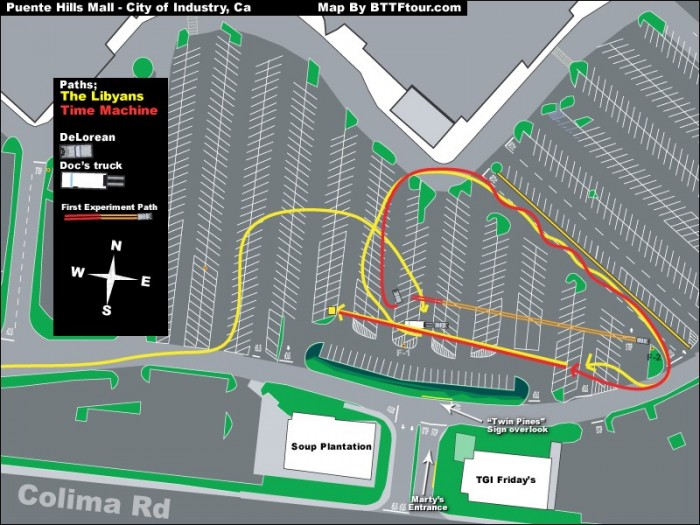# Trivia: 'Back To The Future' Delorean Couldn't Even Get Up To 88MPH At Twin Pines Mall

In a movie that involves time travel and a climax that involves a car harnessing the power of lightning at the exact second that it hits, we don't usually look too hard at the scientific accuracy. Well when it comes to Back to the Future, BullittRide did the math and it turns out that Marty McFly wouldn't have even been able to go back in time in the first place because the Twin Pines Mall would not have enough room to allow the Delorean time machine to get up to the necessary 88 miles per hour.Here are the calculations from Bullitt:

A Delorean will do 0-60mph in 8.8 seconds. To keep things simple I assumed a constant acceleration, up to 60mph this is a reasonable assumption, above 60pmh drag typically starts to take hold and acceleration starts to decrease, so if anything it's an optimistic assumption. I'm also ignoring the fact the the parking lot in the movie was wet, and that the car would have likely been even slower with the added weight from the equipment Doc added to it. And yes, I'm also assuming that Doc hadn't done anything to improve the acceleration performance of the Delorean. The equation for acceleration is A=(V2-V1)/(T2-T1). In this case we know that V1=0, T1=0, V2=60mph, and T2=8.8seconds. Doing a unit conversion we end up with an acceleration of 1.894x10^-3 miles/second^2. So knowing the acceleration and knowing that the car travelled roughly 100 meters or 6.214x10^-2 miles we can look at how long it would have taken to traverse that distance. For an object starting from a standstill the equation for distance assuming a constant acceleration is D=0.5AT^2. Solving that equation for time gives an answer of 8.1 seconds. What that means is that the Delorean wouldn't even be going 60mph (let alone 88mph) by the time it reached Doc and Marty since it takes 8.8 seconds for the car to get to 60mph. Doc wasn't joking about seeing some serious shit... him and Marty would be splattered across the Twin Pines parking lot!

So the Back to the Future Delorean wouldn't have been able to reach 88mph in the Twin Pines Mall (or Lone Pines Mall) parking lot after all, unless of course as Bullitt mentions, Doc could have modified the car to have more acceleration. You can read Bullitt's full calculations here.